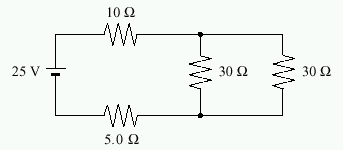# Power dissipated?

## Homework Statement

What is the power dissipated by the 5.0Ω resistor in the following circuit?## Homework Equations

I thought maybe to simplify the resistance like.

1/r=1/30 +1/30
r=15
+10+5
R=30ohms
then find the current
v=ir
25=Ix30
I=.8333
then find power with the 5 ohms?

P=vi
P=25x5
P=75w?

thanks.

You have used the wrong value for voltage.

Remember that only a proportion of the 25V is used in the 5 ohm resistor.

I'll leave it up to you to figure out what the value should be.

Oh, and you've substituded wrongly also....

Last edited:
diazona
Homework Helper
You can also find a formula for power in terms of current and resistance, instead of current and voltage as you have it.

Im trying to think of what the voltage is at that point,
do I use,
EMF=Vab+IR ?
24= vab+0.83*5 or something?

I thought voltage was constant everywhere in a circuit?

Last edited:
The voltage drop across loads connected in parallel is constant(equal to supplied voltage) whereas the voltage drop across loads connected in series is proportional to their resistance (V=IR)

So, calculate the voltage drop across the 5Ohm resistor and use that in you're calculation for Power.

Edit: I got 3.47W as the power dissipation though... I might have missed something.

The voltage drop across loads connected in parallel is constant(equal to supplied voltage) whereas the voltage drop across loads connected in series is proportional to their resistance (V=IR)

So, calculate the voltage drop across the 5Ohm resistor and use that in you're calculation for Power.

Edit: I got 3.47W as the power dissipation though... I might have missed something.

your right its 3.47, the question was split over 2 pages so I saw the wrong answer.

I'm still kind of confused about the voltage when in parallel though.
so if you have a 20v power source that splits 4 ways each is 20v then as far as resistance you treat each on seperatly as if it was a series?

Thanks.

so v=IR
25=Ix30
I=.833333

V=IR
V=.83x5
V=4.15

P=IV
P=4.15x.8333333
P=3.46

Is that right?

Yes, that's how I did it.